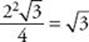﻿ ﻿PRISMS - Solid Geometry - SOLID AND COORDINATE GEOMETRY - SAT SUBJECT TEST MATH LEVEL 1

## SOLID AND COORDINATE GEOMETRY## CHAPTER 12 Solid Geometry### PRISMS

Rectangular solids and cylinders are special cases of geometric solids called prisms. A prism is a three-dimensional figure that has two congruent parallel bases. The (perpendicular) distance between the two bases is called the height. Four prisms are depicted in the figure below.On the Math 1 test, all of the prisms are right prisms, which means that any line segment joining corresponding points on the bases is perpendicular to the bases. In the figure above, the first three prisms are right prisms; the fourth one is not. The volume formulas given in KEY FACTS K1 and K4 are special cases of the following formula.

Key Fact K5

The formula for the volume of any right prism is V = Bh, where B is the area of one of the bases and h is the height.

EXAMPLE 4: What is the volume of the triangular prism below?First calculate the area of a base and multiply it by the height. By KEY FACT H11, the area of an equilateral triangle whose sides are 2 is. So the volume is.

﻿